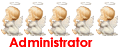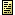本草易讀本草易讀

016-本草易讀.txt [繁體]{請自行解壓縮檔}Re: 本草易讀

<篇名>本草易讀

<目錄>
<篇名>序

，治療疾苦，其效如響。此《傷寒》、《金匱》所由稱方藥之祖也。自唐以降，藥品日增，

，悉折衷于李氏《綱目》，如是而已。蓋本草之撰，代有其作，試舉其目，兼約其略。陶氏

。繼踵者，嘉佑之《補注》；（宋嘉佑間，命郎中林億、禹錫撰《嘉佑補注》）。圖說相謬，
《圖經》繼《補注》而再刊，（宋仁宗命博士蘇洵撰《圖經本草》）。參效者，寇

，（唐縣尉陳藏器撰《本草拾遺》）。不厭僻怪，采百藥而收單方，《証類》複改為《大觀》
（宋時蜀醫唐慎微撰《証類本草》，后改《大觀本草》）。分諸藥而配五行，《補遺》推展

》。）盛矣，李氏《綱目》，（李時珍著《本草綱目》）。統括諸家，引証百氏，萃玉集錦，

<目錄>
<篇名>例

？縱無大功，亦無大過也。茲于小方，則加倍收之，于大劑，則約略舉之。蓋早儲此心于不

，或出殊產異道，失其常性。愚以為用藥之際，當即照本草察其形色，嘗其氣味，各與本草

。或彼方仍不妥，欲另取大方，即照諸方中大方加減取用。仍即所方本部，查其藥性之寒熱

，有以異乎？喜怒憂思，古今之人情，不相侔乎？如果有異而不侔，何《傷寒》、《金匱》諸

<目錄>
<篇名>脈法總括

，浮濡陰虛，浮滑痰熱，浮弦痰飲，浮散危候，浮革勞極，（浮如鼓皮為革）。浮大內虛，多

<目錄>
<篇名>本草易讀卷一

<目錄>本草易讀卷一
<篇名>中風部一

<目錄>本草易讀卷一
<篇名>風寒部二

<目錄>本草易讀卷一
<篇名>溫熱部三

<目錄>本草易讀卷一
<篇名>感冒部四

<目錄>本草易讀卷一
<篇名>瘟疫部五

<目錄>本草易讀卷一
<篇名>傷暑部六

<目錄>本草易讀卷一
<篇名>癱瘓部七

<目錄>本草易讀卷一
<篇名>痿部八

<目錄>本草易讀卷一
<篇名>風濕身痛部九

<目錄>本草易讀卷一
<篇名>如風濕部十

<目錄>本草易讀卷一
<篇名>厥部十一

<目錄>本草易讀卷一
<篇名>脾胃部十二

<目錄>本草易讀卷一
<篇名>酒部十三

<目錄>本草易讀卷一
<篇名>痰飲部十四

<目錄>本草易讀卷一
<篇名>咳嗽部十五Re: 本草易讀

<目錄>本草易讀卷一
<篇名>肺痿部十六

<目錄>本草易讀卷一
<篇名>喘部十七

<目錄>本草易讀卷一
<篇名>瘧部十八

<目錄>本草易讀卷一
<篇名>痢部十九

<目錄>本草易讀卷一
<篇名>瀉部二十

<目錄>本草易讀卷一
<篇名>霍亂部二十一

<目錄>本草易讀卷一
<篇名>轉筋部二十二

<目錄>本草易讀卷一
<篇名>反胃部二十三

<目錄>本草易讀卷一
<篇名>嘔吐部二十四

<目錄>本草易讀卷一
<篇名>脹滿部二十五

<目錄>本草易讀卷一
<篇名>痞堅部二十六Re: 本草易讀

<目錄>本草易讀卷一
<篇名>氣鼓部二十七

<目錄>本草易讀卷一
<篇名>水腫部二十八

<目錄>本草易讀卷一
<篇名>氣部二十九

<目錄>本草易讀卷一
<篇名>癖塊部三十

<目錄>本草易讀卷一
<篇名>諸積部三十一

<目錄>本草易讀卷一
<篇名>黃膽部三十二

<目錄>本草易讀卷一
<篇名>發熱部三十三

<目錄>本草易讀卷一
<篇名>補養部三十四

<目錄>本草易讀卷一
<篇名>勞部三十五

<目錄>本草易讀卷一
<篇名>男陰部三十六

<目錄>本草易讀卷一
<篇名>陽痿部三十七

<目錄>本草易讀卷一
<篇名>諸血部三十八Re: 本草易讀

<目錄>本草易讀卷一
<篇名>諸汗部三十九

<目錄>本草易讀卷一
<篇名>打呃部四十

<目錄>本草易讀卷一
<篇名>麻木部四十一

<目錄>本草易讀卷一
<篇名>癲狂部四十二

<目錄>本草易讀卷一
<篇名>健忘部四十三

<目錄>本草易讀卷一
<篇名>驚悸部四十四

<目錄>本草易讀卷一
<篇名>煩部四十五

<目錄>本草易讀卷一
<篇名>嗜臥部四十六

<目錄>本草易讀卷一
<篇名>小便部四十七

<目錄>本草易讀卷一
<篇名>大便部四十八

<目錄>本草易讀卷一
<篇名>痔漏部四十九

<目錄>本草易讀卷一
<篇名>脫肛部五十

<目錄>本草易讀卷一
<篇名>胡臭部五十一

<目錄>本草易讀卷一
<篇名>虫部五十二

<目錄>本草易讀卷一
<篇名>脹飽部五十三

<目錄>本草易讀卷一
<篇名>失音部五十四

<目錄>本草易讀卷一
<篇名>頭部五十五

<目錄>本草易讀卷一
<篇名>眩暈部五十六

<目錄>本草易讀卷一
<篇名>耳部五十七

<目錄>本草易讀卷一
<篇名>面上斑部五十八Re: 本草易讀

<目錄>本草易讀卷一
<篇名>白屑禿部五十九

<目錄>本草易讀卷一
<篇名>鼻部六十

<目錄>本草易讀卷一
<篇名>口舌部六十一

<目錄>本草易讀卷一
<篇名>唇牙部六十二

<目錄>本草易讀卷二
<篇名>目部六十三

<目錄>本草易讀卷二
<篇名>咽喉部六十四

<目錄>本草易讀卷二
<篇名>瘰瘤部六十五

<目錄>本草易讀卷二
<篇名>心痛部六十六Re: 本草易讀

<目錄>本草易讀卷二
<篇名>腹脅部六十七

<目錄>本草易讀卷二
<篇名>疰病部六十八

<目錄>本草易讀卷二
<篇名>腰腳部六十九

<目錄>本草易讀卷二
<篇名>疝痛部七十

<目錄>本草易讀卷二
<篇名>手足部七十一

<目錄>本草易讀卷二
<篇名>須發部七十二

<目錄>本草易讀卷二
<篇名>渴部七十三

<目錄>本草易讀卷二
<篇名>發斑部七十四

<目錄>本草易讀卷二
<篇名>疹痒疥癬部七十五

<目錄>本草易讀卷二
<篇名>悸部七十六

<目錄>本草易讀卷二
<篇名>瘦部七十七

<目錄>本草易讀卷二
<篇名>膈噎部七十八

<目錄>本草易讀卷二
<篇名>項部七十九

<目錄>本草易讀卷二
<篇名>大風部八十

<目錄>本草易讀卷二
<篇名>卒死部八十一

<目錄>本草易讀卷二
<篇名>危急部八十二

<目錄>本草易讀卷二
<篇名>奇異部八十三

<目錄>本草易讀卷二
<篇名>解毒部八十四Re: 本草易讀

<目錄>本草易讀卷二
<篇名>諸骨哽咽部八十五

<目錄>本草易讀卷二
<篇名>物傷部八十六

<目錄>本草易讀卷二
<篇名>百合部八十七

<目錄>本草易讀卷二
<篇名>湯火傷部八十八

<目錄>本草易讀卷二
<篇名>婦科部八十九

<目錄>本草易讀卷二
<篇名>帶下部九十

<目錄>本草易讀卷二
<篇名>經脈部九十一

<目錄>本草易讀卷二
<篇名>胎漏部九十二

<目錄>本草易讀卷二
<篇名>胎部九十三

<目錄>本草易讀卷二
<篇名>催生部九十四

<目錄>本草易讀卷二
<篇名>胎衣不下部九十五

<目錄>本草易讀卷二
<篇名>去生胎部九十六

<目錄>本草易讀卷二
<篇名>產后部九十七Re: 本草易讀

<目錄>本草易讀卷二
<篇名>婦科一切部九十八

<目錄>本草易讀卷二
<篇名>小兒部九十九

<目錄>本草易讀卷二
<篇名>瘡瘍部一百Re: 本草易讀

<目錄>本草易讀卷二
<篇名>通治部一百零一

<目錄>本草易讀卷二
<篇名>疹痘部一百零二

<目錄>本草易讀卷二
<篇名>金瘡部一百零三

<目錄>本草易讀卷二
<篇名>打跌部一百零四

<目錄>本草易讀卷二
<篇名>內癰部一百零五

<目錄>本草易讀卷二
<篇名>乳部一百零六

<目錄>本草易讀卷二
<篇名>丹毒部一百零七

<目錄>本草易讀卷二
<篇名>附︰手指疔毒

<目錄>本草易讀卷三
<篇名>甘草第一

\x甘遂、海藻。大而緊結者良。\x

\x複脈湯\x 炙草 桂枝 人參 生地 阿膠 麻仁 生姜 大棗 麥冬

\x瀉心湯\x 炙草 大棗 半夏 黃連 黃芩 生姜

\x四逆湯\x 炙草 干姜 附子

\x通脈四逆湯\x 炙草 干姜 附子

\x四逆散\x 炙草 枳實 柴胡 白芍

\x甘草干姜湯\x 炙草 干姜

\x甘草附子湯\x 炙草 白術 附子

\x甘草麻黃湯\x 甘草 麻黃

\x調胃承氣湯\x 炙草 大黃 芒硝

\x甘草湯\x 生甘草

\x甘草蜜粉湯\x 甘草 官粉

\x甘麥大棗湯\x 甘草（二錢） 大棗（七個） 小麥（一把）

\x奔豚湯\x 甘草 半夏 生姜 白芍 當歸 川芎 黃芩 葛根 李根白皮

<目錄>本草易讀卷三
<篇名>黃第二

\x功益大，乃相畏更相使也。\x

。助當歸以生血，佐防風以驅風。生用微涼。實表斂汗者宜之。

，結小尖角。

\x代刀散\x 生 （四錢）穿山甲（一錢） 川芎（三錢） 當歸（二錢） 角刺（一

\x黃 湯\x 黃 白芍 桂枝 苦酒

\x桂枝黃 湯\x

\x五物湯\x 黃 桂枝 白芍 生姜 大棗

\x黃 建中湯\x 桂枝 白芍 炙草 生姜 大棗 膠飴 黃

\x防風黃 湯\x 防風（三錢） 黃 （一兩）

\x玉屏風散\x 防風 黃 白術

\x黃 五物湯\x 黃 白芍 桂枝 生姜 大棗

\x竹葉黃 湯\x 竹葉 黃 生地 白芍 麥冬 台參 當歸 川芎 半夏 黃芩

\x保元湯\x 黃 台參 肉桂 甘草

\x聖愈湯\x 台參 黃 當歸 川芎 白芍 熟地

\x當歸六黃湯\x 當歸 黃 地黃 黃芩 黃連 黃柏

\x黃 建中湯\x 方見上。

<目錄>本草易讀卷三
<篇名>人參第三

\x泰丸參皂同用，是惡而不惡也。療月閉參靈並劑，是畏而不畏也。\x

。民以人參為地方害，不複采取。今所用者，皆是遼參。亦可收子，于十月下種，如種菜法
。秋冬采者堅實，春夏采者虛軟，非地產有虛實也。遼參連皮者，黃潤色如防風，去皮者，

\x歸脾湯\x 人參 元肉 黃 白術 雲苓（同二錢） 當歸（一錢） 棗仁（一

\x理中湯\x 人參 白術 干姜 炙草

\x理中丸\x 方同上。

\x四逆加參湯\x 甘草 干姜 附子 人參

\x新加湯\x 桂枝 白芍 炙草 生姜 大棗 人參

\x通脈四逆湯\x 甘草 干姜 附子

\x人參白虎湯\x 石膏 知母 炙草 粳米 人參

\x小柴胡湯\x 柴胡 黃芩 半夏 人參 炙草 生姜 大棗

\x獨參湯\x 人參

\x參附湯\x 人參 附子

\x生脈散\x 人參 五味 麥冬

\x四君子湯\x 人參 白術 茯苓 炙草

\x異功散\x 台參 白術 雲苓 炙草 陳皮

\x人參白虎湯\xRe: 本草易讀

<目錄>本草易讀卷三
<篇名>沙參第四

<目錄>本草易讀卷三
<篇名>桔梗第五

\x桔梗湯\x（與上驗方第一同。）

\x白散\x 桔梗 貝母 巴豆

\x排膿湯\x 桔梗 甘草 生薑 大棗

\x排膿散\x 桔梗 白芍 枳實

\x中和湯\x 桔梗 白芷 人參 黃 藿香 肉桂 甘草 白朮川芎 當歸 白

\x五苓散\x 茯苓 白朮豬苓 澤瀉 桂枝

<目錄>本草易讀卷三
<篇名>葳蕤第六

\x葳蕤湯\x 葳蕤 石膏 白薇 麻黃 獨活 杏仁 大黃 甘草 青木香

<目錄>本草易讀卷三
<篇名>知母第七

\x白虎湯\x 石膏 知母 粳米 炙草

\x桂枝白虎\x 白虎原方加桂枝。

\x棗仁湯\x 棗仁 甘草 知母 茯苓 川芎

\x大補陰丸\x 知母 黃柏 熟地 龜板

\x虎潛丸\x 龜板 黃柏 知母 熟地 牛膝 白芍 鎖陽 虎骨 當歸 陳皮 羊

\x滋腎丸\x 知母 黃柏 肉桂

<目錄>本草易讀卷三
<篇名>天麻第八

<目錄>本草易讀卷三
<篇名>白術第九

，祛勞倦，消痰水，除肌熱。君黃芩而安胎，佐枳實以消痞。血燥無濕者禁用，瘡瘍潰后忌

，上有毛。方莖，莖端有花，淡紫碧紅數色，根作椏生。大塊紫花，根如雲頭者佳。蓋古方

\x桂附去桂加白湯\x 甘草 生薑 大棗 附子 白朮

\x越婢加術湯\x 麻黃 甘草 石膏 薑 棗 白朮

\x麻黃加術湯\x 麻黃 杏仁 炙草 桂枝 白朮

\x理中丸\x 人參 白朮乾薑 甘草

\x白術散\x 白朮川椒 川芎 牡蠣

\x茯苓飲\x 茯苓 人參 陳皮 白朮枳實 生薑

\x桂苓甘術湯\x 桂枝 茯苓 白朮甘草

\x木香丸\x 木香 青皮 白朮薑黃 豆蔻 阿魏（等分）

<目錄>本草易讀卷三
<篇名>蒼術第十

\x平胃散\x 蒼術 陳皮 濃朴 炙草

\x越鞠湯、丸\x 蒼術 香附 山梔 撫芎 神曲

\x萬靈丹\x 蒼術（八兩） 麻黃 羌活 荊芥 防風 細辛 川烏 草烏 川芎 石

\x流精湯\x 蒼術（二錢） 山藥（五錢） 茱萸肉（二錢） 車前子（三錢） 雲

\x萬應丸\x（即痧藥） 蒼術（三兩，焙） 丁香（六錢，公母俱可） 麝香（三分）

（三兩。）

<目錄>本草易讀卷三
<篇名>狗脊十一

<目錄>本草易讀卷三
<篇名>貫眾十二

<目錄>本草易讀卷三
<篇名>遠志十三

<目錄>本草易讀卷三
<篇名>淫羊藿十四

<目錄>本草易讀卷三
<篇名>巴戟天十五

<目錄>本草易讀卷三
<篇名>肉蓯蓉十六

<目錄>本草易讀卷三
<篇名>鎖陽十七

<目錄>本草易讀卷三
<篇名>元參十八

<目錄>本草易讀卷三
<篇名>地榆十九

。七月開花紫黑色。根外黑裡紅似柳根。

<目錄>本草易讀卷三
<篇名>丹參二十

，除煩熱。止腸鳴腹痛，理崩帶症瘕。功同四物，為女科要藥。Re: 本草易讀

<目錄>本草易讀卷三
<篇名>紫參二十一

\x紫參湯\x 紫參 甘草

<目錄>本草易讀卷三
<篇名>紫草二十二

，除心腹邪氣。大便滑者忌之。

<目錄>本草易讀卷三
<篇名>白頭翁二十三

\x白頭翁湯\x 白翁 黃連 黃柏 秦皮

<目錄>本草易讀卷三
<篇名>白芨二十四

<目錄>本草易讀卷三
<篇名>三七二十五

<目錄>本草易讀卷三
<篇名>黃連二十六

\x惡菊花、元參、鮮皮、芫花、僵蠶，畏冬花、牛膝，勝烏頭，解巴豆毒。忌豬肉，惡冷\x
\x水。\x

（第四。）

\x香連丸\x 黃連 木香

\x赴筵散\x 黃連 乾薑

\x黃連湯\x 黃連 桂枝 炙草 人參 半夏 薑 棗

\x黃連阿膠湯\x 黃連 阿膠 黃芩 白芍 雞子黃

\x黃連粉\x 黃連為末。

<目錄>本草易讀卷三
<篇名>胡連二十七

<目錄>本草易讀卷三
<篇名>黃芩二十八

\x胎。山茱萸、龍骨為使，惡蔥實，畏丹砂、藜蘆。\x

，類紫草。亦有獨莖者，葉細長青色，兩兩相對，六月開紫花。中枯而飄者名枯芩，即今所

\x黃芩湯\x 黃芩 白芍 甘草 大棗（各三錢）

（諸方第一。）

<目錄>本草易讀卷三
<篇名>秦艽二十九

<目錄>本草易讀卷三
<篇名>柴胡三十

，產后忌之。

\x小柴胡湯\x 柴胡 黃芩 半夏 人參 炙草 生薑 大棗

\x大柴胡湯\x 柴胡 黃芩 半夏 白芍 枳實 大黃 薑 棗

\x清肝湯\x

<目錄>本草易讀卷三
<篇名>前胡三十一

<目錄>本草易讀卷三
<篇名>防風三十二

\x畏萆 ，殺附子毒，惡藜蘆、白蘞、乾薑、芫花。\x

，白者不堪用。

<目錄>本草易讀卷三
<篇名>獨活三十三

（第四。）

<目錄>本草易讀卷三
<篇名>羌活三十四

，利周身百節之痛。血虛頭痛，二活並忌。

\x九味羌活湯\x 羌活 防風 蒼術 川芎 細辛 白芷 當歸 黃芩 甘草 薑

<目錄>本草易讀卷三
<篇名>升麻三十五

，隨決隨生，不治數日必死，瘥后斑點彌歲方減，此惡毒之氣所致也。蜜煎升麻，時時食之
，並用水煎升麻綿拭洗之。（驗方第一。）

\x升麻鱉甲湯\x 升麻 鱉甲 甘草 當歸 雄黃 蜀椒

<目錄>本草易讀卷三
<篇名>苦參三十六

<目錄>本草易讀卷三
<篇名>白鮮皮三十七

，莖青，葉稍白，如槐，亦似茱萸。四月開花淡紫色。根似小蔓荊，皮黃白而心實。

<目錄>本草易讀卷三
<篇名>元胡三十八Re: 本草易讀

<目錄>本草易讀卷三
<篇名>貝母三十九

、江陵府、郢、壽、隨、鄭、蔡、潤、滁州皆有之，以川產者為良。貝母中有獨顆團不作兩

<目錄>本草易讀卷三
<篇名>山慈姑四十

（第三。）
\x紫金錠\x 淨慈姑（二兩，焙淨） 五倍子（二兩，焙） 千金子（去油，一兩） 麝

、死牛馬等毒，並用涼水磨服一錠，或吐或利即愈。癰疽發背，疔腫楊梅，一切惡瘡，風疹

<目錄>本草易讀卷三
<篇名>茅根四十一

<目錄>本草易讀卷三
<篇名>龍膽草四十二

，療時氣之溫熱。骨間寒熱之郁，肝家驚癇之邪，赤睛赤肉之突，熱痢熱狂之火。消癰瘍而

<目錄>本草易讀卷三
<篇名>細辛四十三

\x石，反藜蘆。\x

，兼治睫倒，通 鼻氣塞，亦解耳聾。不可過用。

<目錄>本草易讀卷三
<篇名>白薇四十四

<目錄>本草易讀卷三
<篇名>白前四十五

（第二。）

<目錄>本草易讀卷三
<篇名>當歸四十六

，止諸般臟腑疼痛。溫瘧癖痢之苦，痿痺症瘕之邪。排膿止痛，養血生肌。諸風諸氣諸勞悉

\x當歸四逆湯\x 當歸 白芍 桂枝（各三錢） 細辛 通草 甘草（各二錢） 大

\x當歸生薑羊肉湯\x 當歸（三兩） 生薑（五兩） 羊肉（一斤）

\x當歸芍藥湯\x 當歸（三） 白芍（斤） 川芎（三） 白術（四） 茯苓（四） 澤

\x當歸貝母苦參丸\x 當歸 貝母 苦參（等分）

\x當歸散\x 當歸 白芍 川芎 黃芩 白術（等分）

\x當歸\x 白芍 棗仁（炒） 茯神 麥冬 辰砂 生地 甘草

\x鎮心丸\x 辰砂（五錢） 琥珀（五錢） 天竺黃（五錢） 膽星（一兩） 牛黃
（三錢） 雄黃（二錢） 珍

<目錄>本草易讀卷三
<篇名>芎四十七

\x出天台山者為台芎；出江南者為撫芎。\x

，作叢而莖細。其葉倍香，名蘼蕪是也。七八月間開碎白花。時珍曰︰蜀地少寒，人多栽蒔
，深秋莖葉亦不萎也。

（宜用十服，諸方第一。）

<目錄>本草易讀卷三
<篇名>蛇床子四十八

。在處有之，以揚州、襄州者為良。

<目錄>本草易讀卷三
<篇名>本四十九

，一切風濕。粉刺酒 之疾，癰疽疥癬之毒。君木香除霧露之襲沿，同白芷去頭面之垢屑。

<目錄>本草易讀卷三
<篇名>白芷五十

\x沖和膏\x 紫荊皮（五） 獨活（三） 白芷（三） 赤芍（二） 菖蒲（二）

<目錄>本草易讀卷三
<篇名>白芍五十一

\x藜蘆。\x

，除煩退熱。固腠理而斂汗，和血脈而收氣，解腹痛而平肝，除后重而止痢。心痞脅痛之疾
，鼻衄目澀之 ，癰腫疝瘕之凝，痔漏瘡疥之科。平肺脹之喘逆，伸足攣之拘急。婦科一切

，有紅白紫淡數種。

<目錄>本草易讀卷三\白芍五十一
<篇名>赤芍

\x桂枝加芍湯\x 桂枝原方倍白芍。

\x芍藥甘草湯\x 白芍 甘草

\x芍藥甘草附子湯\x

\x桂枝去芍湯\x 桂枝原方去白芍。

<目錄>本草易讀卷三
<篇名>丹皮五十二Re: 本草易讀

<目錄>本草易讀卷三
<篇名>木香五十三

<目錄>本草易讀卷三
<篇名>甘松香五十四

<目錄>本草易讀卷三
<篇名>山柰五十五

<目錄>本草易讀卷三
<篇名>良薑五十六

<目錄>本草易讀卷三\良薑五十六
<篇名>紅豆蔻

<目錄>本草易讀卷四
<篇名>草豆蔻五十七

<目錄>本草易讀卷四
<篇名>白豆蔻五十八

<目錄>本草易讀卷四
<篇名>砂仁五十九

，乃補益脾胃之靈丹。

<目錄>本草易讀卷四
<篇名>益智仁六十

<目錄>本草易讀卷四
<篇名>蓽茇六十一

<目錄>本草易讀卷四
<篇名>肉豆蔻六十二

，駐小兒之乳霍。療心腹之脹痛，兼療虫痛，調赤白之下痢，兼調氣痢。霍亂中惡之疾，痰

，惟烘乾密封，則稍可留。

<目錄>本草易讀卷四
<篇名>破故紙六十三

\x故紙丸\x 故紙（四兩） 菟絲子（四兩） 胡桃肉（一兩） 乳香 沒藥（各錢）

\x養血還精丸\x 故紙（二兩） 茯苓（一兩） 沒藥（五錢）

<目錄>本草易讀卷四
<篇名>薑黃六十四

<目錄>本草易讀卷四
<篇名>郁金六十五

\x白金丸\x 郁金（七兩） 白礬（三兩）

<目錄>本草易讀卷四
<篇名>莪術六十六

<目錄>本草易讀卷四
<篇名>三棱六十七

\x三棱煎\x 三棱（一斤）

<目錄>本草易讀卷四
<篇名>香附六十八

\x九氣湯\x 香附 郁金 甘草 薑引 水治九種心痛，膈氣、風氣、寒氣、憂氣、

\x黃鶴丹\x 香附（一斤） 黃連（半斤）

\x青囊丸\x 香附（一斤） 烏藥（五兩）

\x一品丸\x 香附（焙末）

\x快氣湯\x 香附（一斤） 砂仁（半斤） 炙草（四兩）

\x小烏沉湯\x 香附（二十兩） 烏藥（十兩） 甘草（一兩）

\x艾附丸\x 香附（三兩） 艾葉（半兩）

\x四製香附丸\x

\x五香丸\x 靈脂（一斤） 香附（一斤） 黑丑（二兩） 白丑（二兩）

<目錄>本草易讀卷四
<篇名>排草香六十九

<目錄>本草易讀卷四
<篇名>藿香七十

\x藿香正氣散\x 藿香 蘇葉 雲苓 白芷 檳榔 濃朴 陳皮 桔梗 半夏 炙草

<目錄>本草易讀卷四
<篇名>澤蘭七十一

<目錄>本草易讀卷四
<篇名>香薷七十二

\x香薷飲\x 香薷（一斤） 濃朴（半斤） 白扁豆（半斤）

\x香薷煎\x 香薷（十斤）

\x薷術丸\x 薷葉（一斤）

<目錄>本草易讀卷四
<篇名>荊芥莖穗七十三

，背脊筋骨之痛。

\x愈風散\x 芥穗

<目錄>本草易讀卷四
<篇名>薄荷莖葉七十四Re: 本草易讀

<目錄>本草易讀卷四
<篇名>龍腦薄荷七十五

，對節生。氣甚辛烈。六七月開花成穗，如蘇穗，水紅色，穗中有細子，狀如荊芥子，可種
，宿根亦自出。

\x龍腦雞蘇丸\x 水蘇（一斤） 生地（六兩） 黃芩 蒲黃 麥冬 阿膠 台參 木

<目錄>本草易讀卷四
<篇名>紫蘇梗葉七十六

<目錄>本草易讀卷四\紫蘇梗葉七十六
<篇名>蘇子

\x蘇子降氣湯\x 蘇子 陳皮 濃朴 前胡 肉桂 半夏 當歸 甘草 薑 棗

<目錄>本草易讀卷四
<篇名>甘菊七十七

<目錄>本草易讀卷四
<篇名>艾葉七十八

（第四。）

（十二。）

\x膠艾湯\x 阿膠 艾葉 川芎 甘草 當歸 地黃 白芍

\x艾薑丸\x 艾葉 乾薑

<目錄>本草易讀卷四
<篇名>茵陳七十九

\x茵陳蒿湯\x 茵陳 山梔 大黃

\x茵陳五苓散\x 五苓散（五分） 加茵陳（十分）

\x茵陳羹\x

<目錄>本草易讀卷四
<篇名>青蒿八十

\x根無使莖。四件皆同使，翻然成痼疾。\x

<目錄>本草易讀卷四
<篇名>益母草八十一

<目錄>本草易讀卷四\益母草八十一
<篇名>益母子

<目錄>本草易讀卷四
<篇名>夏枯草八十二

<目錄>本草易讀卷四
<篇名>寄奴草八十三

<目錄>本草易讀卷四
<篇名>旋覆花八十四

\x金沸草湯\x 沸草（二兩） 蔥白（十四枚） 新絳（少許）

\x旋覆代赭湯\x 旋覆（三兩） 代赭石（一兩） 甘草（三兩） 參（二兩） 薑 棗

<目錄>本草易讀卷四
<篇名>青葙子八十五

，與雞冠花一樣。

<目錄>本草易讀卷四
<篇名>雞冠八十六

，儼如青葙之穗；扁卷而平大者，儼如雄雞之冠。花大有圍一二尺者，層層卷出可愛，子在

<目錄>本草易讀卷四
<篇名>紅花八十七

\x紅花酒\x

<目錄>本草易讀卷四
<篇名>胭脂八十八

\x胭脂膏\x

<目錄>本草易讀卷四
<篇名>大小薊八十九

。善消瘀血，尤能安胎。小薊力微，破瘀生新，保精養血。又退熱補虛，開胃下食。解胸膈

<目錄>本草易讀卷四
<篇名>續斷九十

<目錄>本草易讀卷四
<篇名>漏蘆九十一

<目錄>本草易讀卷四
<篇名>苧根九十二

<目錄>本草易讀卷四
<篇名>大青九十三

<目錄>本草易讀卷四
<篇名>胡蘆巴九十四

\x沉香內消丸\x 沉香 木香 蘆巴 小茴

<目錄>本草易讀卷四
<篇名>馬藺子九十五

，調婦科產后血運。經脈不止之疾，崩中帶下之 。根葉略同。

<目錄>本草易讀卷四
<篇名>牛蒡子九十六

<目錄>本草易讀卷四\牛蒡子九十六
<篇名>牛蒡根葉

<目錄>本草易讀卷四
<篇名>蒼耳九十七

<目錄>本草易讀卷四\蒼耳九十七
<篇名>莖葉

\x萬應膏\x 蒼耳連莖根葉，水熬去渣，再熬成膏。或貼或含化，或酒下。治一切癰